# Thread: Partial integration help

1. ## Partial integration help

Howdy,

So, i have a question about partial integration.

Acctually this question concerns Integration in general.

How do you know how to choose the variables for substitution?

For example i have $\displaystyle \int x^2 cos(\pi x) dx$

If we pick U =$\displaystyle x^2$ and V = $\displaystyle -\frac{1}{\pi}*sin(\pi x)$
dU = $\displaystyle 2x$ and dV = $\displaystyle cos(\pi x)$

so...

$\displaystyle UV -\int V*dU$
= $\displaystyle \frac{1}{\pi}sin(\pi x) * x^2 -\int 2x * -\frac{1}{\pi}sin(\pi x)$

...
This is the point where i start to doubt wether i've choosen the right variables for substitution.

2.Originally Posted by JonesHowdy,

So, i have a question about partial integration.

Acctually this question concerns Integration in general.

How do you know how to choose the variables for substitution?

For example i have $\displaystyle \int x^2 cos(\pi x) dx$

If we pick U =$\displaystyle x^2$ and V = $\displaystyle -\frac{1}{\pi}*sin(\pi x)$
dU = $\displaystyle 2x$ and dV = $\displaystyle cos(\pi x)$

so...

$\displaystyle UV -\int V*dU$
= $\displaystyle \frac{1}{\pi}sin(\pi x) * x^2 -\int 2x * -\frac{1}{\pi}sin(\pi x)$

...
This is the point where i start to doubt wether i've choosen the right variables for substitution.
You picked right! If the new integral is harder than the one you started with you picked wrong. Notice that the new integral has the power of x reduced by one (this is good). Now do parts again.

3.Originally Posted by JonesHowdy,

So, i have a question about partial integration.
"Integration by parts", not "partial integration".

Acctually this question concerns Integration in general.

How do you know how to choose the variables for substitution?

For example i have $\displaystyle \int x^2 cos(\pi x) dx$
You try both ways. Obviously if you are going to go from u to du and from dv to v, you will want to choose u to be easily differentiable and dv to easily integrable. Also, you will then need to integrate vdu so you want that to be simpler than "udv". Here, $\displaystyle x^2$ and $\displaystyle cos(\pi x)$ are easily both differentiable and integrable. So you look at "udv".

If you try $\displaystyle u= x^2$ and $\displaystyle dv= cos(\pi x)dx$ you have du= 2xdx and $\displaystyle v= \frac{1}{\pi}sin(\pi x)$ so $\displaystyle udv= \frac{2}{\pi}x sin(\pi x)$. Yes, that's easier because the power of x has been reduced. I think I would try integration by parts again!

If you try u= cos(x) and $\displaystyle dv= x^2dx$ you have $\displaystyle dv= -sin(x)$ and $\displaystyle v= \frac{1}{3}x^3$ so that $\displaystyle vdu= -\frac{1}{3}x^3sin(x)$. Power of x has gone up one- more complicated!

If we pick U =$\displaystyle x^2$ and V = $\displaystyle -\frac{1}{\pi}*sin(\pi x)$
dU = $\displaystyle 2x$ and dV = $\displaystyle cos(\pi x)$

so...

$\displaystyle UV -\int V*dU$
= $\displaystyle \frac{1}{\pi}sin(\pi x) * x^2 -\int 2x * -\frac{1}{\pi}sin(\pi x)$

...
This is the point where i start to doubt wether i've choosen the right variables for substitution.

4. L.I.A.T.E. (logs, inverse trigs, algebra, trigs, exponentials) Integration by parts - Wikipedia, the free encyclopedia seems a handy thing.

However, if a certain amount of trial and error is inevitable, then, with no disrespect to the honorable tradition of 'vdu and udv', I find it can help to work directly with the expressions thus denoted. Then the choice is a matter of depicting the product-rule with 'legs crossed or uncrossed'.The challenge of integration by parts is simply to fill out one of these shapes usefully, but starting in one of the bottom corners instead of (as happens in differentiation) the top.

As has been pointed out, you were right on track, because...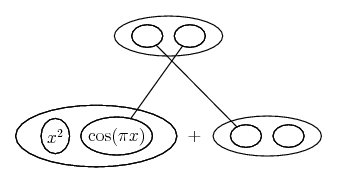leads to...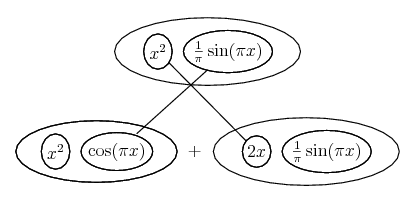... the only problem, that the lower level is no longer equal to what we wanted to integrate...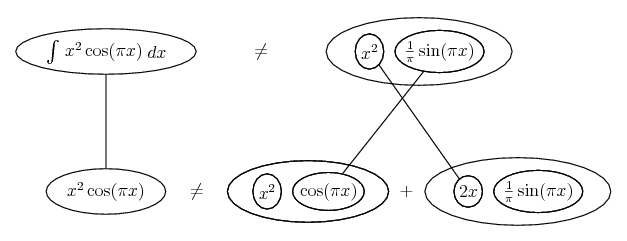But fix that...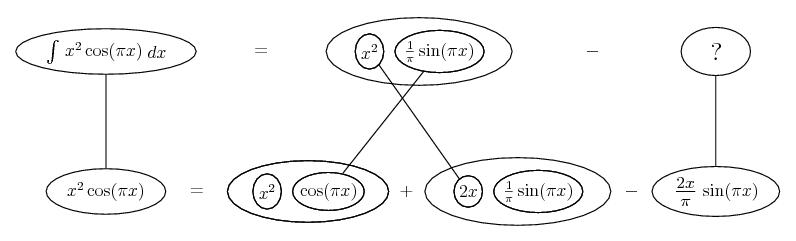Now we face a problem similar to the first one, so it's a case of 'integration by parts twice'...... or cutting out the l.h.s. for a bit more space...Speaking for myself, if I take the wrong road of...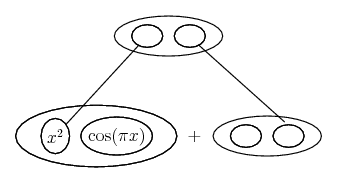... leading to ...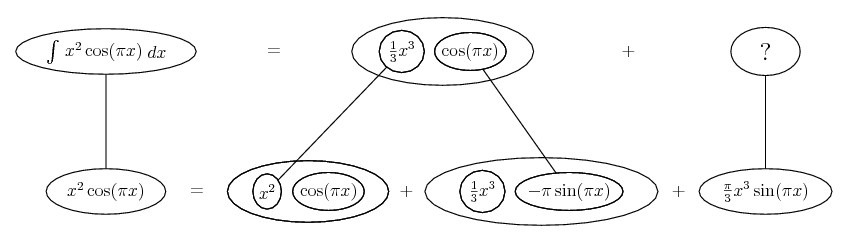... then I'm much better able to review and re-attack than if I'm juggling u's and v's. (OR... I'll use a diagram to help me re-assign them.)

Don't integrate - balloontegrate!

Balloon Calculus Forum

#### Search Tags

integration, partial